# 1200 screws

1200 screws are to be divided into 3 groups so that in the 1st group there are 300 screws more than in the 2nd group and in the 2nd group 150 screws less than in the 3rd group. How many screws will be in each group?

a =  550
b =  250
c =  400

### Step-by-step explanation:

a+b+c=1200
a = 300+b
b = c-150

a+b+c = 1200
a-b = 300
b-c = -150

a = 550
b = 250
c = 400

Our linear equations calculator calculates it.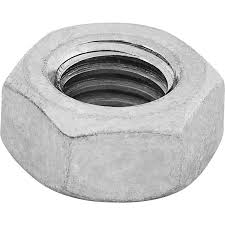Did you find an error or inaccuracy? Feel free to write us. Thank you!Tips to related online calculators
Do you have a system of equations and looking for calculator system of linear equations?

## Related math problems and questions:

• 600 pencils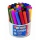600 pencils we want to be divided into three groups. The biggest groups have ten pens more than the smallest. How many ways can this be done?
• Mixture of coffee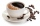They have to prepare a mixture of coffee in packing centres so that 1 kg costs 240 CZK. There are two types of coffee in stock at the price of 220 CZK per 1 kg and 300 CZK per 1 kg. How many kilograms of each species need to be mixed to prepare 50 kg of t
• Boys and money270 USD boys divided so that Peter got three times more than Paul and Ivan has 120 USD more than Paul. How much each received?
• Three workshopsThere are 2743 people working in three workshops. In the second workshop works 140 people more than in the first and in third works 4.2 times more than the second one. How many people work in each workshop?
• Four pupilsFour pupils divided \$ 1485 so that the second received 50% less than the first, the third 1/2 less than a fourth, and fourth \$ 154 less than the first. How much money had each of them?
• Three friends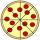Divide 570 euros to three friends so that first will get 50 euros less than the second and third twice more than the first. How many euros will get everyone?Divide number 135 into two additions so that one adds 30 more than 2/5 of the other add. Write the bigger one.
• Circus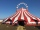On the circus performance was 150 people. Men were 10 less than women and children 50 more than adults. How many children were in the circus?
• School year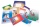At the beginning of the school year, 396 notebooks and 252 textbooks are ready to be distributed in the classroom. All pupils receive the same number of notebooks and the same amount of textbooks. How many pupils are there in the class if you know that th
• The nuts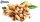There are 159 nuts in two bags, 15 more in the first than in the second, how many nuts are in the bags?
• MegapizzaMegapizza will be divided among 100 people. First gets 1%, 2nd 2% of the remainder, 3rd 3% of the remainder, etc. Last 100th 100% of the remainder. Which person got the biggest portion?
• GroupA group of kids wanted to ride. When the children were divided into groups of 3 children, one remain. When divided into groups of 4 children, 1 remains. When divided into groups of 6 children, 1 remains. After divided into groups of 5 children, no one lef
• Pears and orangesThere are 3 times as many pears as oranges. If a group of children receives 5 oranges each, there will be no oranges left over. If the same group of children receives 8 pears each, there will be 21 pears leftover. How many children and oranges are there?
• Two hundred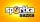Two hundred ten athletes competed in three athletics races on three fields. One hundred five athletes competed in the first, 60 in the second, and everyone else in the third. On the individual courts, the athletes were divided into groups. Each group, alt
• Three workers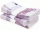The three workers received € 2,850 together for the work done. They divided them according to the time worked so that the first received 20% less than the second and the third € 50 more than the second. How much EUR did each worker receive?
• Factory and divisionsThe factory consists of three auxiliary divisions total of 2,406 employees. The second division has 76 employees less than the 1st division, and the 3rd division has 212 employees more than the 2nd. How many employees have each division?
• EurosPeter, Jane, and Thomas have together € 550. Tomas has 20 euros more than Jane, Peter € 150 less than Thomas. Determine how much has each of them.